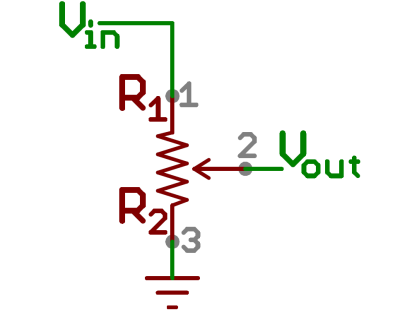## Conductive slime

This is an experiment of using water and flour to create this conductive slime as a tangible control. To acheive this, think of the slime as a potentiometer,  simply read(analogRead Pin) the voltage change of the point where you moved,  then mapping it to the height of the graph.

In this video, the p5 sketch will morph in the corresponding direction of how I shape the slime.

An equivalent comparison of the diagram:（I should use a voltage divider so that I only need two wires :)  )